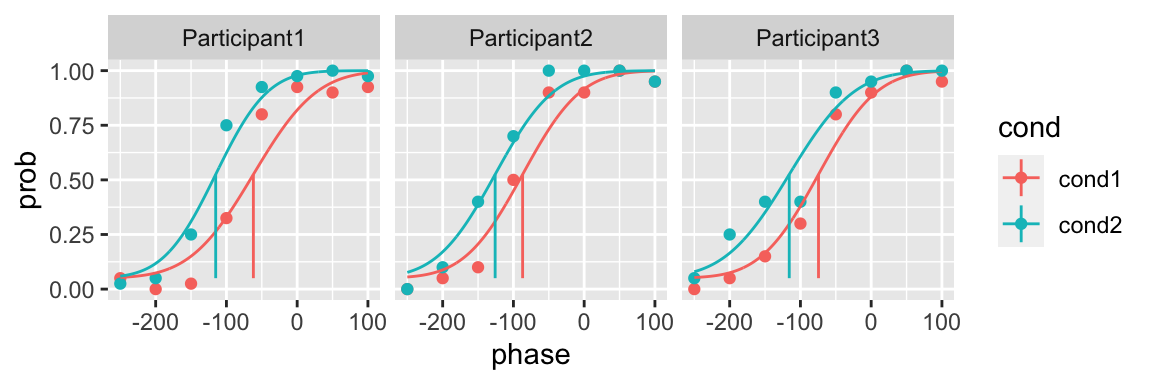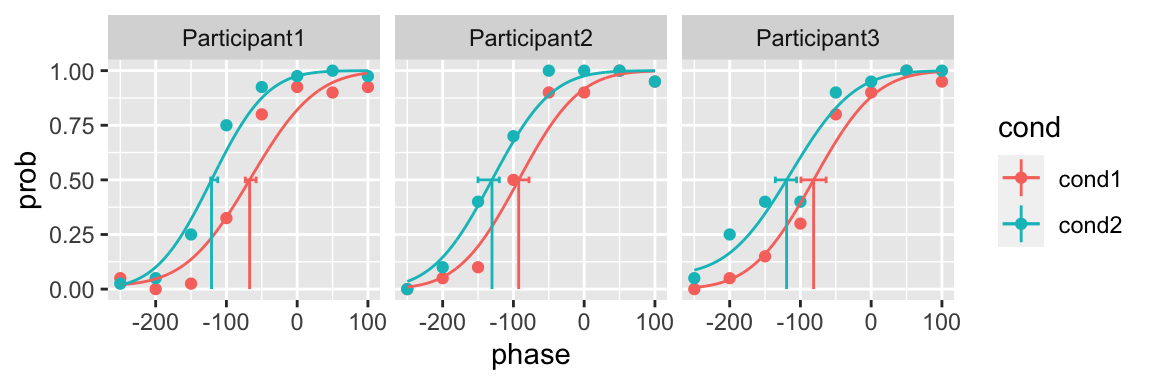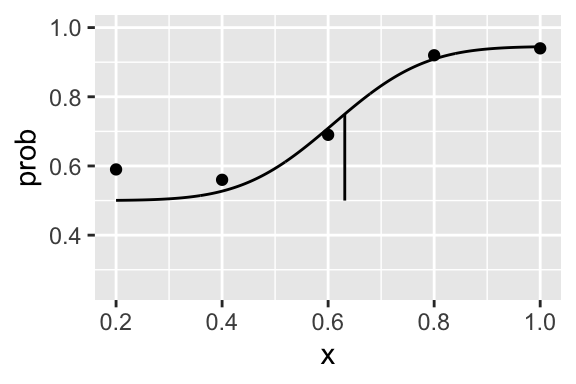The guess argument of quickpsy allows you to set the leftward asymptote of the psychometric function.

The default value is 0, but it can be changed:

library(quickpsy)
fit <- quickpsy(qpdat, phase, resp, grouping = c("participant", "cond"),
guess = 0.05, bootstrap = "none")

plot(fit, color = cond)The leftward asymptote can also be free as a parameter (it wouldn’t be a guess rate in this case)

fit <- quickpsy(qpdat, phase, resp, grouping = c("participant", "cond"),
guess = TRUE, B = 10) # only 10 bootstrap samples

plot(fit, color = cond)The lapses argument of quickpsy works as the guess argument.

We can combined guesses and lapses. For example, for a task with a fixed guess rate of 50% and a variable lapse rate parameter we can do


library(ggplot2)

n <- 100
x <- c(.2, .4, .6, .8, 1)
k <- c(59, 56, 69, 92, 94)
y <- k / n
dat <- data.frame(x, k, y)

fit <- quickpsy(dat, x, k, n, guess = 0.5, lapses = TRUE, bootstrap = "none")

plot(fit) + ylim(0.25, 1)Observe that the lapse rate parameter is now the p3 parameter (p1 and p2 correspond to the position and scale parameters respectively)

fit\$par
#> # A tibble: 3 x 2
#>   parn     par
#>   <chr>  <dbl>
#> 1 p1    0.610
#> 2 p2    0.136
#> 3 p3    0.0545

If both the leftward and the rightward asymptotes are free, they will appear as the p3 and p4 parameters respectively.